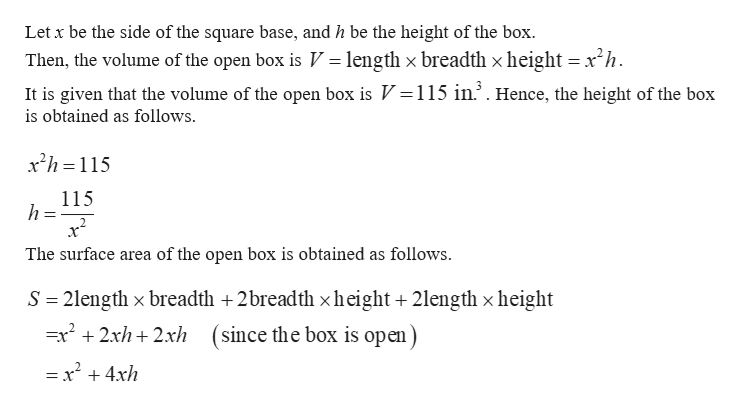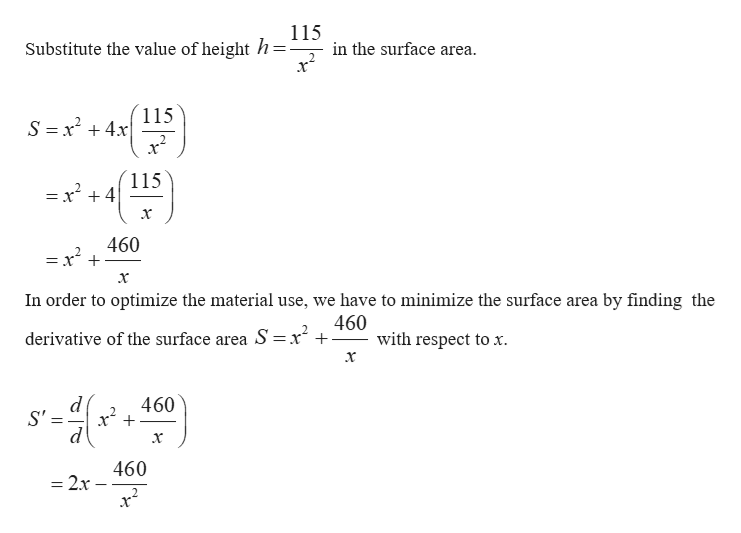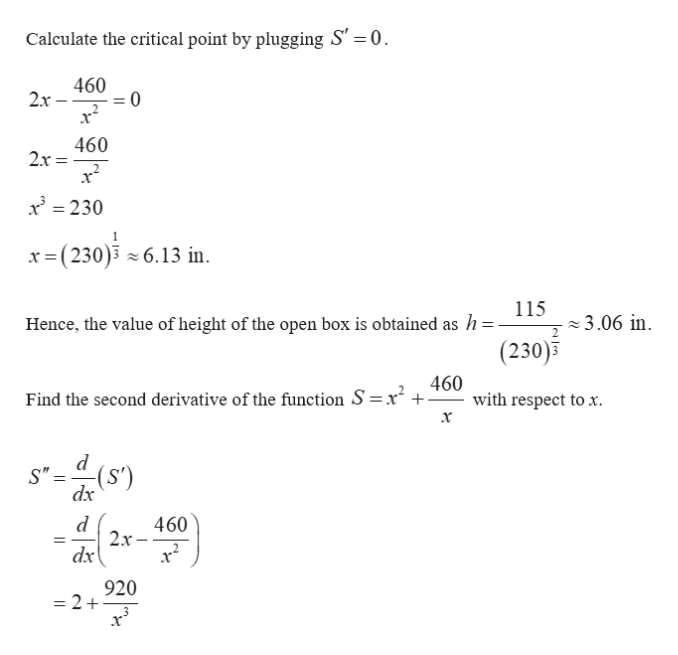# If an open box has a square base and a volume of 115 in.3 and is constructed from a tin sheet, find the dimensions of the box, assuming a minimum amount of material is used in its construction. (Round your answers to two decimal places.)

Question

If an open box has a square base and a volume of 115 in.3 and is constructed from a tin sheet, find the dimensions of the box, assuming a minimum amount of material is used in its construction. (Round your answers to two decimal places.)

check_circle

Step 1help_outlineImage TranscriptioncloseLet x be the side of the square base, and h be the height of the box. Then, the volume of the open box is V length x breadth x height x2h It is given that the volume of the open box is V 115 in.2. Hence, the height of the box is obtained as follows x2h 115 115 2 The surface area of the open box is obtained as follows 2length x breadth +2breadth x height +2length x height (since the box is open S =x2 2xh2xh = x2 4xh fullscreen
Step 2help_outlineImage Transcriptionclose115 Substitute the value of height h= in the surface area. 2 x 115 S =x24x 115 = x +4 460 =x x In order to optimize the material use, we have to minimize the surface area by finding the 460 with respect to x derivative of the surface area S =x d S' 460 460 = 2x fullscreen
Step 3help_outlineImage Transcriptionclose0 Calculate the critical point by plugging S 460 2x 0 460 2x x230 x (230) 6.13 in 115 3.06 in Hence, the value of height of the open box is obtained as h (230) 460 with respect to x. x Find the second derivative of the function S x P S"= (s) dx 2x- dx 920 -2+ fullscreen

### Want to see the full answer?

See Solution

#### Want to see this answer and more?

Solutions are written by subject experts who are available 24/7. Questions are typically answered within 1 hour.*

See Solution
*Response times may vary by subject and question.
Tagged in

### Other